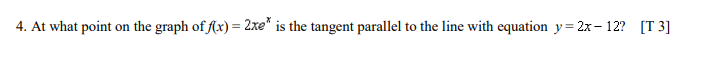# Question Solved1 Answer4. At what point on the graph of f(x) = 2xe* is the tangent parallel to the line with equation y=2x- 12? [T 3]Transcribed Image Text: 4. At what point on the graph of f(x) = 2xe* is the tangent parallel to the line with equation y=2x- 12? [T 3]
More
Transcribed Image Text: 4. At what point on the graph of f(x) = 2xe* is the tangent parallel to the line with equation y=2x- 12? [T 3]